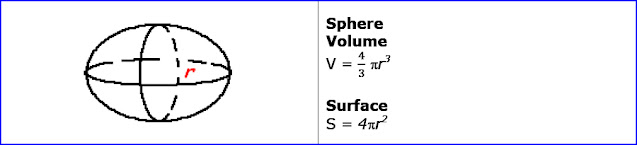# C++ Exercises

## C++ Program to calculate the volume and surface of the sphere.

Let see the program to calculate the volume and surface of the sphere.

```
Example:

Formula:sphere

```
```
/* to calculate the volume of the sphere. */
#include <iostream>

using namespace std;
int main()
{

cout<<"volume of sphere = "<<volume<<" units."<<endl;

return 0;
}

```
##### Output:
```

volume of sphere = 1265.62 units.

```
```
/* to calculate the surface of the sphere. */
#include <iostream>

using namespace std;
#include <math.h>
int main()
{

```